Get instant live expert help with Excel or Google Sheets“My Excelchat expert helped me in less than 20 minutes, saving me what would have been 5 hours of work!”

#### Post your problem and you’ll get Expert help in seconds.

Your message must be at least 40 characters
Our professional Expert are available now. Your privacy is guaranteed.

# How to Get the Top Level Domain (TLD) from Data in Excel

The Top Level Domain (TLD) refers to the last part of the full domain name or URL that follows after the dot “.” symbol, like .com, .net, .org etc. We can extract the top level domain from the full domain name or email address using a formula that consists of several text functions, like RIGHT, LED, FIND and SUBSTITUTE functions.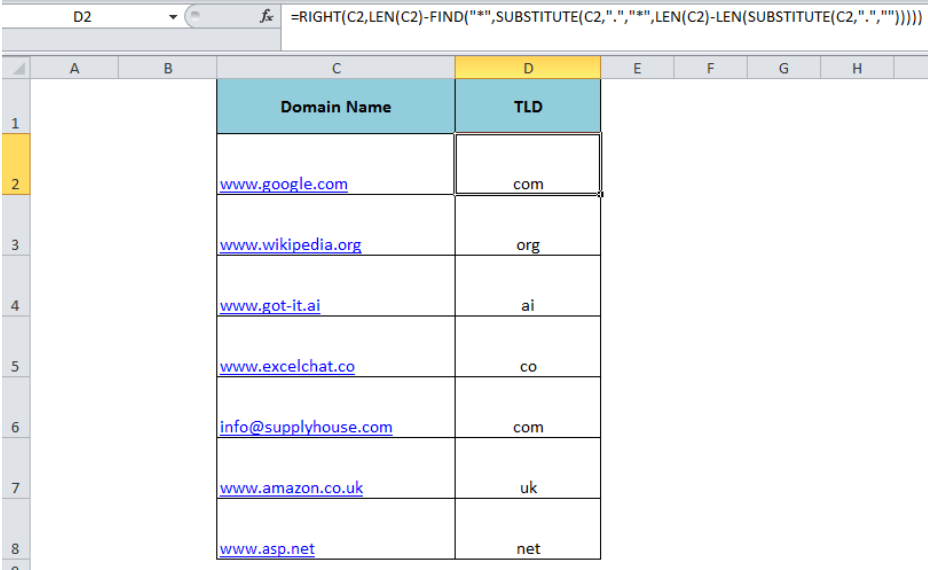Figure 1. Extract Top Level Domain From URL

## Formula Syntax

The generic formula syntax is:

`=RIGHT(domain,LEN(domain)-FIND("*",SUBSTITUTE(domain,".","*",LEN(domain)-LEN(SUBSTITUTE(domain,".","")))))`

Here “domain” refers to the full domain name or URL or email address as a cell reference or text value directly entered in this formula syntax. Suppose we have a list of domains containing URLs in column C and want to get the top level domain (TLD) from the full domain name in column D. As per the formula syntax, we need to use the following formula in cell D2;

`=RIGHT(C2,LEN(C2)-FIND("*",SUBSTITUTE(C2,".","*",LEN(C2)-LEN(SUBSTITUTE(C2,".","")))))`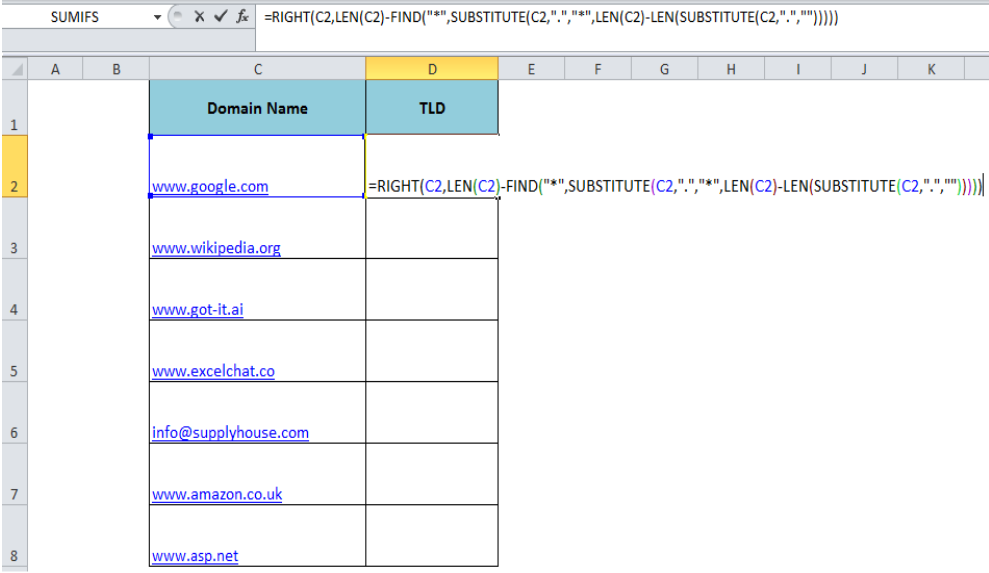Figure 2. The Formula to Extract Top Level Domain From URL

## Working of the Formula

In this formula, we have used the RIGHT function to extract the number of characters from the right side of the domain name. The following part of the formula returns the number of characters in the RIGHT function as per the location of the last dot “.” position in the URL string;

`LEN(C2)-FIND("*",SUBSTITUTE(C2,".","*",LEN(C2)-LEN(SUBSTITUTE(C2,".",""))))`

In order to locate the position of last “dot” symbol in the string, we need to first replace the last “dot” symbol with asterisk “*” in the following formula part, and then FIND function returns the location of the asterisk in the string;

`SUBSTITUTE(C2,".","*",LEN(C2)-LEN(SUBSTITUTE(C2,".","")))`

We can select this formula part in the formula bar and press the F9 key to see the result.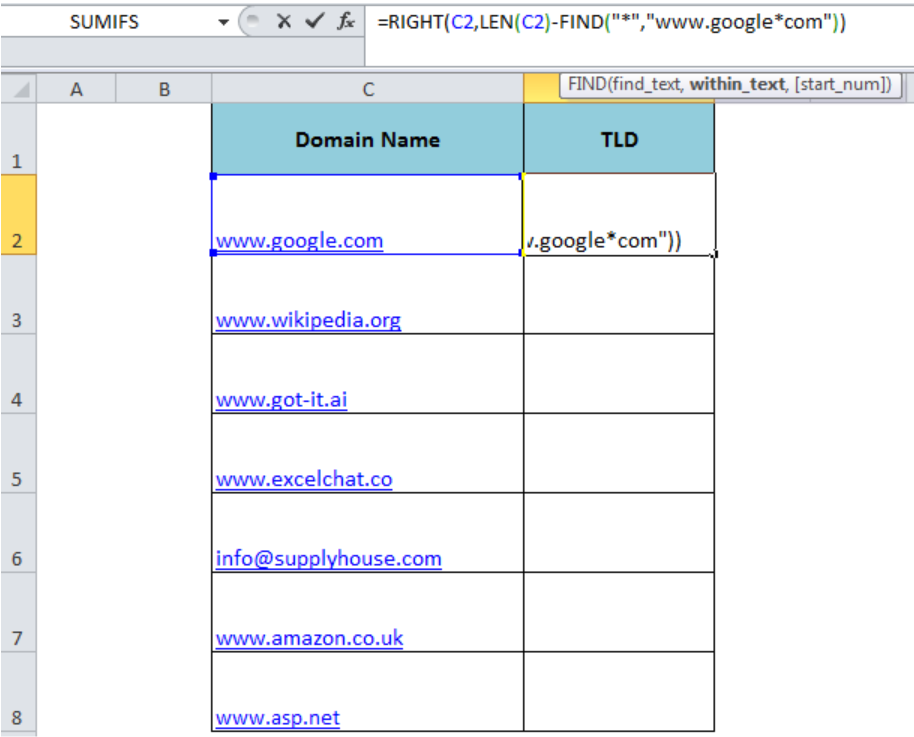Figure 3. Replacing the Last Dot Symbol With Asterisk

Then the FIND function returns the position of the asterisk to calculate the number of characters required to extract from the full domain name. The following formula part calculates the number of characters by subtracting the position of the asterisk symbol from the total characters of the domain;

`LEN(C2)-FIND("*","www.google*com")`

As the total characters’ length in cell C2 is 14 and FIND function returns the position of asterisk symbol at 11th character, so the resulting number of characters would be 3 (14-11) to feed into the RIGHT function. Select the above part of the formula and press F9 again to see the result.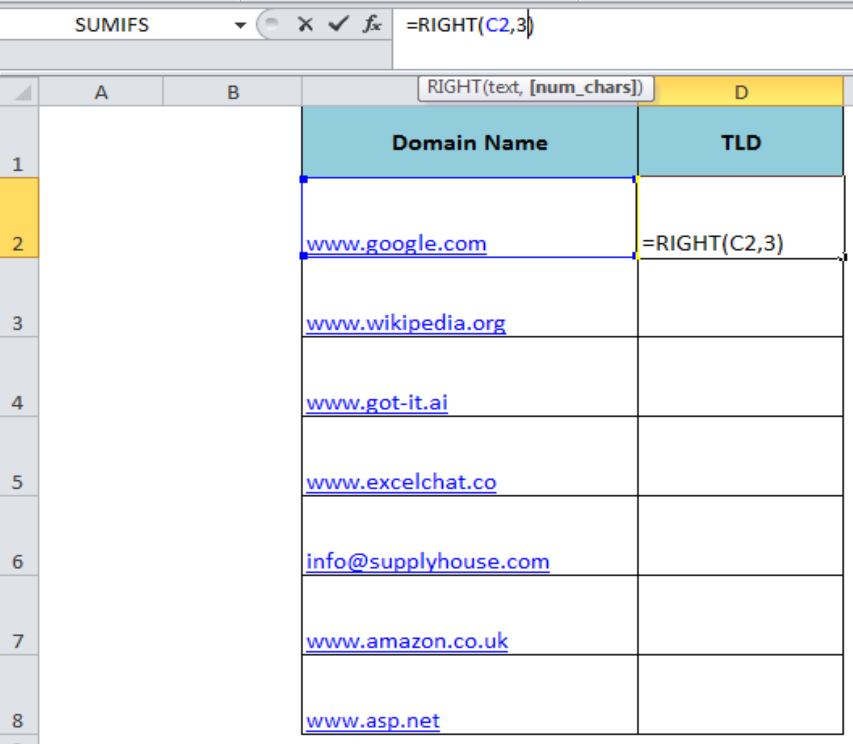Figure 4. Calculating the Number of Characters Required

Finally, the RIGHT function extracts the number of characters from the right side of the domain name return by the remaining part of the formula. Press Enter and copy the formula down to other cells if required.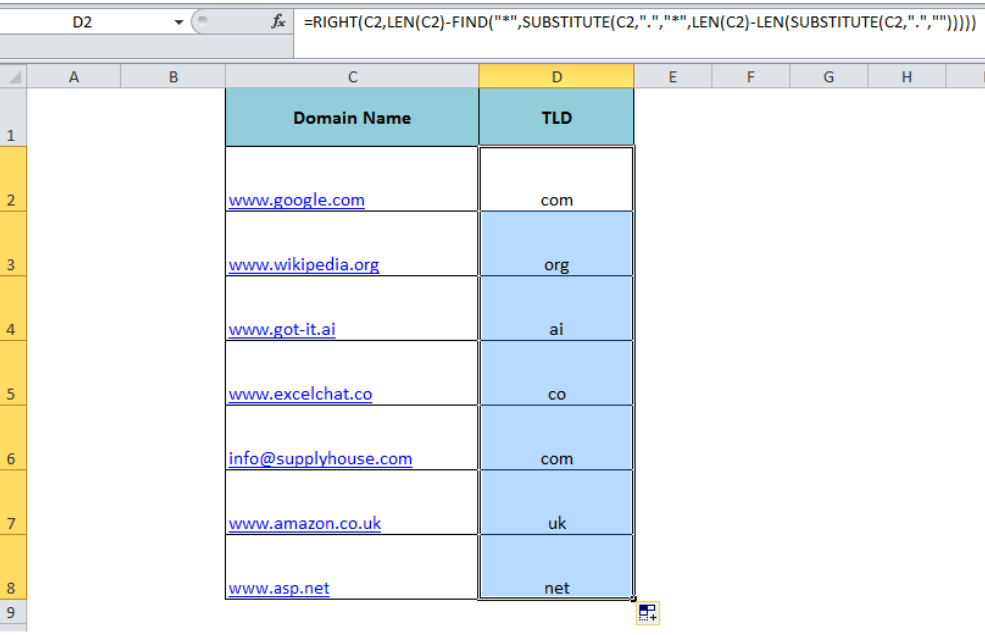Figure 5. Final Preview of Formula Result

## Instant Connection to an Expert through our Excelchat Service

Most of the time, the problem you will need to solve will be more complex than a simple application of a formula or function. If you want to save hours of research and frustration, try our live Excelchat service! Our Excel Experts are available 24/7 to answer any Excel question you may have. We guarantee a connection within 30 seconds and a customized solution within 20 minutes.

### Did this post not answer your question? Get a solution from connecting with the expert.Another blog reader asked this question today on Excelchat:
Solution examplesI am having issues creating the one way data table to show the means and standard deviations when you change the proportion of portfolio 1. I have the mean formula and the standard deviation formula at the top and -1 through 1 down the left side. I highlight all of it and create a data table. I reference the "proportion of portfolio 1" cell in the column input box but when the data table comes out it has all the same numbers all they way down the table.
Solved by G. Q. in 49 minsI am trying to figure out how to use a sumif on SUBSTITUTE(A2,"*","~*") where A2 is a formula with 3371-4
Solved by M. Y. in 19 minsI have 2 columns one of them with a dropdown list of 20 and the other one with 2 options only. I want that when Comment A and B are chosen in the first column then the secon column populate "X" STRAIGHT AWAY. HOW DO i DO IT?
Solved by I. D. in 10 minsWhat formula do I use to create a running balance across several worksheets as the numbers continue to change? For example. I have created a drop down list for every month of the year from Jan - Dec for people to sign up for sessions. As they sign up for sessions, I want to create a list beside their names to show how many sessions they have signed up for. So if they sign up for 6 the summary should automatically display 6, if they sign up for 1 more it would instantly show 7 and so on for each person? Make sense
Solved by C. F. in 59 minsWhat is the formula for splitting up one column text into 2 columns?
Solved by B. H. in 20 mins## Subscribe to Excelchat.coAnother blog reader asked this question today on Excelchat: